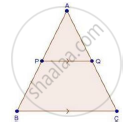Share
Notifications

View all notifications
Books Shortlist
Your shortlist is empty

# Solution for In Abc, P Divides the Side Ab Such that Ap : Pb = 1 : 2. Q is a Point in Ac Such that Pq || Bc. Find the Ratio of the Areas of triangle Apq and Trapezium Bpqc. - CBSE Class 10 - Mathematics

Login
Create free account

Forgot password?

#### Question

In ABC, P divides the side AB such that AP : PB = 1 : 2. Q is a point in AC such that PQ || BC. Find the ratio of the areas of ΔAPQ and trapezium BPQC.

#### SolutionWe have,

PQ || BC

And "AP"/"PB"=1/2

In ΔAPQ and ΔABC

∠A = ∠A [Common]

∠APQ = ∠B [Corresponding angles]

Then, ΔAPQ ~ΔABC [By AA similarity]

By area of similar triangle theorem

("area"(triangleAPQ))/("area"(triangleABC))="AP"^2/"AB"^2

rArr("area"(triangleAPQ))/(("area"(triangleAPQ)+"area"("trap.BPQC")))=1^2/3^2   ["AP"/"PB"=1/2]

⇒ 9ar (APQ) = ar(ΔAPQ) + ar(trap. BPQC)

⇒ 9ar (APQ) − ar(ΔAPQ) = ar(trap. BPQC)

⇒ 8ar(APQ) = ar(trap. BPQC)

rArr("area"(triangleAPQ))/("area"("trap.BPQC"))=1/8

Is there an error in this question or solution?

#### Video TutorialsVIEW ALL 

Solution In Abc, P Divides the Side Ab Such that Ap : Pb = 1 : 2. Q is a Point in Ac Such that Pq || Bc. Find the Ratio of the Areas of triangle Apq and Trapezium Bpqc. Concept: Areas of Similar Triangles.
S